### Test: College Algebra

Quadratic equations appear often in physics. The basic kinematic equations for the positionof a particle as a function of time, with an initial velocity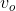(a constant) and constant accelerationcan be written as,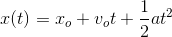This is a quadratic function in. The function therefore gives the position as a quadratic function of time. If we are dealing with a free-falling object under Earth's gravitational field, we might write this function in the form,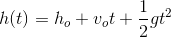to express the "height"of the object at a given timefalling with a constant acceleration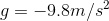. Here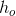the initial height (a constant). The units for acceleration are meters-per-square second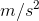. The negative acceleration is a convention to signify that the direction of the acceleration is downward.

 1 Find the time required for a ball dropped from a height of 100 m from rest to reach the ground using the quadratic function for height written below,(Hint, what is the value of the height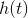when the ball strikes the ground?).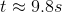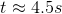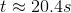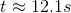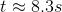1/1 questions

0%

## Access results and powerful study features!

Take 15 seconds to create an account.